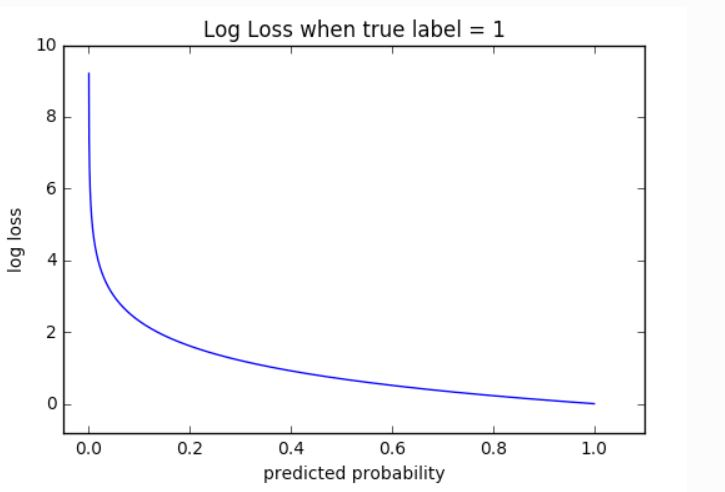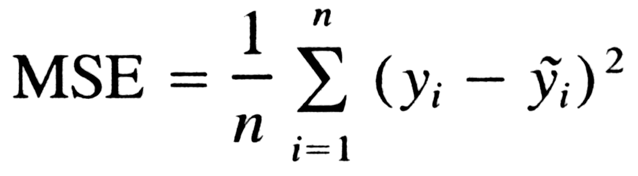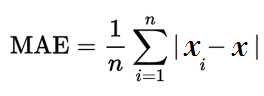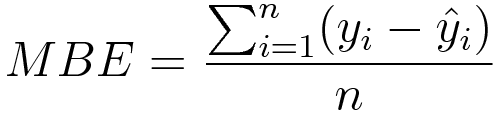• Category
• >Deep Learning
• >Machine Learning

# What Are Different Loss Functions Used as Optimizers in Neural Networks?

• Rohit Dwivedi
• Jun 17, 2020
• Updated on: Jan 19, 2021The final goal in Machine Learning is to increase or decrease the “Objective function”. The loss function is used to measure how good or bad the model is performing. It is used to compute to estimate the prediction given by the model in terms of generalizability.

For example, we have to identify the dog from a set of dog images. There are more than 100 images of dogs and cats that are mixed in the dataset. For each dog picture, the label that is associated is ‘1’ and the picture has no dog present as ‘0’. To solve the problem these images are fed into the network that reverts a floating number through which it is predicted that which class the images are related to either 0 or 1. If the outcome is 1 then there is a dog present and vice versa.

But Neural Networks gives us the real number as outcomes such as 0.1, 0.7, and 0.8. And from these sets of numbers, it is identified whether 0.1 belongs to the dog or not. Evidently, 0.8 is closer to 1, so if the output is 0.8, the probability is that it is a dog as compared to 0.5. But there would be cases when even the return probability 0.5 or even 0.1 is a dog. Yes, there is a concept of back-propagation for tuning parameters. But before this, the validation techniques need to correct the result with the actual result. In this type of scenario, Loss functions come into the picture.

Sometimes the activation results become senseless without systematic validation. Also, there is no fixed loss function that can be used in all places. These loss functions depend on a variety of different factors.

## Different types of Loss Functions

Loss functions are mainly classified into two different categories that are Classification loss and Regression Loss. Classification loss is the case where the aim is to predict the output from the different categorical values for example, if we have a dataset of handwritten images and the digit is to be predicted that lies between (0-9), in these kinds of scenarios classification loss is used.

Whereas if the problem is regression like predicting the continuous values for example, if need to predict the weather conditions or predicting the prices of houses on the basis of some features. In this type of case, Regression Loss is used.

## Classification Losses

• ### Cross-Entropy Loss / Log Loss

It computes the performance of classification tasks where results lie between probability values 0 and 1. As the predicted probability disunites from the true label, the cross-entropy loss gets increased. Log loss of 0 is considered to be a perfect model. Both cross-entropy and log loss are a bit different from each other but when we are computing errors between 0 and 1, they result in the same thing.

Check here to know more about cross-entropy loss or log loss.Cross-Entropy loss /Log Loss

• ### Hinge Loss

Another loss for binary classification task is the hinge loss function which was initially developed to use with the support vector machine models. It is recommended to be used where the target labels are in (-1,1) in binary classification tasks. Hinge loss makes the examples have the right sign, allocating more error when there is dissimilarity in the sign of the true label and predicted label.

Check here to know more about Hinge Loss.Hinge Loss

• ### Square Loss

Hinge loss has many different additional losses. A famous loss is squared hinge loss simply computes the square of the score hinge loss. It makes the error in numerical making it easier to work with and smoothens the error. If hinge loss does not give better efficiency then there are chances that square loss might give you reliable performance.Square Loss

Check here more about Square Loss. There are other losses also which you can read like focal loss, logistic loss, and an exponential loss here.

## Regression Losses

• ### Mean Square Loss / L2 Loss

It is more often used regression loss that is computed by taking the average squared difference between actual and predicted observations. It mainly takes into consideration the average magnitude of error ignoring the direction. Due to squaring the predictions that are distant from the true values are penalized laboriously in comparison to less diverged predictions. It is easy to compute gradients because of the mathematical properties there in L2 Loss.Mean Squared Error

• ### Mean Absolute Error

It is computed by taking the average of the sum of absolute differences between the true and predicted variables. Similar to MSE it also calculates magnitude ignoring the direction. It is tough to compute the gradients in MAE as there is a need for linear programming also MAE does not use square so it is more strong to outliers.Mean Absolute Error

• ### Mean Bias Error

It is not that often used loss in regressions. MBE is almost similar to MSE, the only difference that makes them different is that absolute values are not taken here. It is less used but it can be used to check if the model has a negative bias or positive bias.Mean Bias Error

You can check Keras documentation of Loss Function where different probabilistic loss function and regression losses are given with their explanation. You can check here for the documentation.

## Conclusion

It is very important to check if your model is able to generalize or not. For this purpose, we make use of the loss function so as to check the performance of the model, how good and how bad the model is performing.

In this blog, I have discussed what loss functions and different types of loss function used for Classification as well as Regression problems in predictive modeling. Also, there are many other different losses that are used to compute error which can be checked on Keras Documentation, where a variety of loss functions are given and discussed that are used in different scenarios.